## ORIGINAL RESEARCH article

Front. Comput. Neurosci., 29 September 2011
https://doi.org/10.3389/fncom.2011.00038

# Frequency-dependent changes in NMDAR-dependent synaptic plasticity

• 1 Bernstein Center Freiburg, University of Freiburg, Freiburg, Germany
• 2 Neurobiology and Biophysics, University of Freiburg, Freiburg, Germany
• 3 Department of Physics and Astronomy, University of California Los Angeles, Los Angeles, CA, USA
• 4 Department of Neurology, University of California Los Angeles, Los Angeles, CA, USA
• 5 Department of Neurobiology, University of California Los Angeles, Los Angeles, CA, USA

The NMDAR-dependent synaptic plasticity is thought to mediate several forms of learning, and can be induced by spike trains containing a small number of spikes occurring with varying rates and timing, as well as with oscillations. We computed the influence of these variables on the plasticity induced at a single NMDAR containing synapse using a reduced model that was analytically tractable, and these findings were confirmed using detailed, multi-compartment model. In addition to explaining diverse experimental results about the rate and timing dependence of synaptic plasticity, the model made several novel and testable predictions. We found that there was a preferred frequency for inducing long-term potentiation (LTP) such that higher frequency stimuli induced lesser LTP, decreasing as 1/f when the number of spikes in the stimulus was kept fixed. Among other things, the preferred frequency for inducing LTP varied as a function of the distance of the synapse from the soma. In fact, same stimulation frequencies could induce LTP or long-term depression depending on the dendritic location of the synapse. Next, we found that rhythmic stimuli induced greater plasticity then irregular stimuli. Furthermore, brief bursts of spikes significantly expanded the timing dependence of plasticity. Finally, we found that in the ∼5–15-Hz frequency range both rate- and timing-dependent plasticity mechanisms work synergistically to render the synaptic plasticity most sensitive to spike timing. These findings provide computational evidence that oscillations can have a profound influence on the plasticity of an NMDAR-dependent synapse, and show a novel role for the dendritic morphology in this process.

## Introduction

Most experimental studies on synaptic plasticity have used one of two protocols: a rate-based protocol or a timing based protocol. A wealth of rate-based in vitro experiments have shown that sustained low frequency stimulation of presynaptic afferents induces long-term depression (LTD; Dudek and Bear, 1992), while high-frequency stimulation generates long-term potentiation (LTP) of synaptic strengths (Bliss and Lomo, 1973; Stevens and Sullivan, 1998; Malenka and Bear, 2004). Theoretically, this phenomenon can be captured by a bidirectional, activity-dependent plasticity rule (Bienenstock et al., 1982; Lisman, 1989). These experiments and theories have mostly focused on the asymptotic values of synaptic strengths, where additional stimuli do not induce more LTP or LTD.

However, during behavioral tasks, neurons typically fire only a small number of spikes, which can induce synaptic plasticity in vitro (Dobrunz and Stevens, 1999) but are unlikely to saturate the synaptic strengths (Dudek and Bear, 1992; Petersen et al., 1998; Zhang et al., 2009). Further, behavioral data show that a significant amount of learning can occur within a few trials. Consistently, incremental and rapid changes in hippocampal place cells’ firing, hypothesized to occur due to synaptic plasticity, have been observed after only about a dozen spikes per neuron (Mehta et al., 1997, 2000). These studies support the hypothesis that during learning, synaptic strengths evolve incrementally in response to a small number of spikes, and thus operate in an unsaturated state. In fact, saturation of synaptic strengths may limit the encoding and learning capability of the network (Moser et al., 1998). Further, NMDAR-dependent synaptic plasticity is strongly modulated by precise spike timing (spike timing-dependent plasticity – STDP; Magee and Johnston, 1997; Markram et al., 1997; Nishiyama et al., 2000; Bi and Poo, 2001; Sjöström et al., 2001; Wittenberg and Wang, 2006; Zhang et al., 2009). Typical STDP induction protocols keep the spike rate fixed, whereas in natural spike patterns both spike rate and timing vary simultaneously.

Therefore, to understand the role of synaptic plasticity in learning, it is important to characterize the evolution of synaptic strengths in the sub-saturating regime where both spike rate and timing vary. Here, we do so by using a commonly employed model of synaptic plasticity based on postsynaptic calcium influx (Artola and Singer, 1993; Cummings et al., 1996; Karmarkar and Buonomano, 2002; Shouval et al., 2002, 2010; Abarbanel et al., 2003). Notably, this widely studied model makes several novel predictions about the role of oscillations and bursting in the induction of synaptic plasticity when the effects of short-lasting spike patterns are considered. Specifically, we show that when the number of spikes used to induce plasticity was kept fixed, the amount of LTP was a non-monotonic function of firing rate, and at firing rates above ∼30 Hz the number of spikes in the stimulus affected the amount of plasticity produced. These findings were obtained using a reduced model that could be solved analytically. The findings were further confirmed using more realistic, stochastic synapses as well as using a detailed, multi-compartment model of a CA1 pyramidal neuron. The results obtained using these different methods agree which shows that our results are mathematically and biologically sound. Using the detailed neuron model we further show that the dendritic location of a synapse can have a profound impact on plasticity such that it can switch the sign of plasticity because of changes in the dendritic distance-dependent amplitude of the back-propagating action potential. The model suggests that spike bursts can switch a network from rate-based learning to spike timing based learning. These results provide a novel cellular mechanism for how different rhythms and spike patterns may influence plasticity and learning (Sivan and Kopell, 2004). Our results imply that oscillations may differentially modulate the plasticity induced by top-down versus bottom-up influences (Buschman and Miller, 2007) in the neocortex, and by direct versus indirect inputs to the hippocampus (Colgin et al., 2009). Additionally, this may reduce interference between multiple memories during learning (Fusi and Abbott, 2007).

## Materials and Methods

We simulated a synapse undergoing NMDAR-mediated postsynaptic calcium-dependent plasticity. Consistent with a large body of evidence, in this model of synaptic plasticity we made three main assumptions:

(1) Both LTD and LTP are determined by the amplitude and duration of postsynaptic calcium (Clement-Cormier et al., 1980; Dudek and Bear, 1992; Yang et al., 1999; Nishiyama et al., 2000; Johnston et al., 2003).

(2) The amount of synaptic weight change is proportional to the duration of calcium transients until saturation is reached (Dudek and Bear, 1992; Petersen et al., 1998; Mizuno et al., 2001).

(3) The rate of change in synaptic strength as a function of calcium concentration has an upper limit (Artola and Singer, 1993; Nishiyama et al., 2000; Shouval et al., 2002; Johnston et al., 2003). This assumption is relaxed for the results shown in Figure 6.

With these assumptions, we investigated the rate and timing dependence of plasticity induced by a few tens of spikes as is observed in natural spike patterns during behavior in vivo.

In its simplest form, the membrane potential at the synapse located on the postsynaptic side can be described as a linear sum of excitatory postsynaptic potentials [EPSP(t)] and the back-propagating action potential [BPAP(t); Shouval et al., 2002, 2010; Cai et al., 2007], if the somatic membrane depolarization is above spiking threshold, as described in Eq. 1: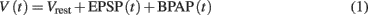where Vrest = −65 mV is the resting potential of the neuron. In plasticity experiments, typically the postsynaptic neuron is forced to spike by external current injection; thus, the BPAP is always present during the stimulation protocol. We modeled EPSP and BPAP using separate differential equations, because BPAP propagation and amplitude are determined by the dynamics of voltage gated K+ and Na+ channels which are much faster than the sub-threshold, passive mechanisms that govern the EPSP dynamics, especially for the weak synapses considered here. For the rare cases when the membrane potential is depolarized (≥ −50 mV), or when the synapses are strong enough to activate voltage-dependent Na+ and K+ dependent currents, the simplified model described in Eq. 1has to be revised. Further, non-linear effects such as boosting of EPSPs due to BPAP are not included in this simple model.

### Synaptic Inputs

The temporal evolution of the synaptic input EPSP(t), which is composed of both AMPAR- and NMDAR-mediated currents is given by the following: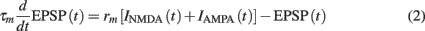where τm = 20 ms is the membrane time constant, and rm is the membrane resistance, set to unity. The two synaptic currents are given by: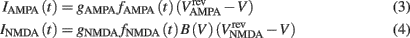where gAMPA, gNMDA (=0.1295, 1.295 μS) are the channels’ conductances,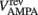,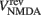(=0, 0 mV) are the reversal potentials of their AMPA and NMDA currents. gAMPA, gNMDA describe the effective current (mediated by Na+ and K+ ions) flowing through the two channels. The magnesium block, described by B(V), is defined below (Eq. 7). fAMPA, fNMDA are the AMPA and NMDA activation functions respectively, with an exponential form: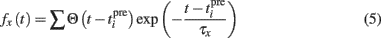where Θ is the step function, Θ(x) = 1 if x > 0 and Θ(x) = 0 otherwise,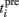is the time of the ith presynaptic activation, x = (AMPA, NMDA) and τAMPA, τNMDA (=2, 40 ms) are the respective time constants.

The NMDA receptor mediated calcium influx is given by: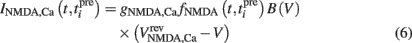Here, the reversal potential for calcium influx is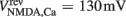. The value of the NMDA-dependent calcium conductance gNMDA,Ca was varied across different simulations (cf. Results). This calcium influx also contributes to the synaptic current in addition to the NMDA and AMPA currents given in Eqs 3 and 4.

B(V) describes the voltage-dependent relief of the NMDAR blockage by magnesium ions (Jahr and Stevens, 1990):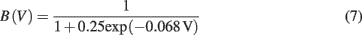The BPAP was modeled as:The BPAP had a peak amplitude of A = 100 mV at time tpost, and subsequently decayed as a sum of two exponentials: 70% of the BPAP decayed rapidly (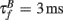), and the remaining represented the slow after-depolarization (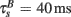; Magee and Johnston, 1997). The frequency-dependent dynamics of the BPAP are described below (Eqs 10–12).

For simplicity, we assumed that the NMDARs are the only sources of plasticity-inducing calcium. Therefore, the postsynaptic calcium concentration [Ca] was given by an ordinary differential equation (Eq. 6). The Ca influx decayed with a time constant τCa = 25 ms (Bloodgood and Sabatini, 2005) as: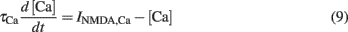Additional sources of calcium can be readily incorporated into the model, however they were not included in this study. Notably, the main conclusions drawn in this paper are qualitatively independent of the particular calcium source modeled. Further, we did not explicitly model calcium buffering or the spread of calcium in the spine. However, we assumed that calcium spreads in the spine, and becomes available to plasticity processes, instantaneously.

### Frequency-Dependent Synaptic Depression and Facilitation

Here we used a stochastic model of frequency-dependent synaptic depression and facilitation that does not distinguish between the pre- and postsynaptic mechanisms (Markram et al., 1998; Cai et al., 2007). Thus, although we describe the short-term plasticity in terms of the dynamics of synaptic vesicles, it applies equally to postsynaptic receptors. We assumed that a synapse has Nmax total vesicles and that of those N are available for release. Each of the N vesicles can be released with a probability p, however only one vesicle can be released for a presynaptic spike. This gives a synaptic transmission probability P = 1 − (1 − p)N. When a vesicle is released, N is decreased by one, thus reducing the synaptic transmission probability P. Each vesicle has a recovery time constant (τrec), and a vesicle is replenished with an independent and constant probability per unit time, 1/τrec. Here we used Nmax = 3, N = 1, p = 0.16, and τrec = 500 ms (Cai et al., 2007). We used the same stochastic model to model synaptic facilitation, except in this case, upon arrival of a presynaptic spike the vesicle release probability was increased by a constant factor α. That is, after a spike the new release probability pnew was changed from the previous release probability (pprev) according to the following relationship: pnew = pprev + αp. This increased release probability recovered to the original value exponentially with a fixed time constant, τF. Here we used α = 0.8 and τF = 100 ms (Cai et al., 2007).

### Frequency-Dependent Depression of BPAP

We used a phenomenological description of frequency-dependent depression of BPAP based on the idea of an activity-dependent usage of membrane resources. This is similar to the model of frequency-dependent depression of synaptic amplitude used above, developed by Markram et al. (1998). To model the activity-dependent BPAP amplitude depression we scaled the BPAP amplitude by a factor y (Eq. 11) that evolves in the following way: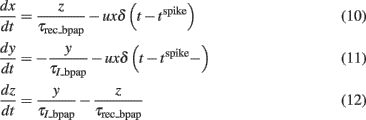Where x, y, and z are the fractions of membrane resources that are important for maintenance of the BPAP amplitude, i.e., the availability of Na+ channels (Colbert et al., 1997; Stuart et al., 1997; Gasparini, 2011) in the recovered, active and inactive states, respectively. Note that x, y, and z are phenomenological parameters. τrec_bpap is the recovery time constant from depression and τI_bpap is the inactivation time constant (Eqs 11 and 12). δ(ttspike) = 1 if (ttspike) = 0 and δ(ttspike) = 0 otherwise. The Eqs 10–12 describe how the resource variable y is used by each spike at time tspike. For activity-dependent depression of BPAP we kept the variable u which describes the effective use of the resources, constant. Here, we used τI_bpap = 50 ms, τrec_bpap = 20 ms to approximately fit the experimentally observed kinetics of BPAP depression (Gordon et al., 2006).

### Calcium Concentration-Dependent Change in EPSP Amplitude

The synaptic weight w was updated according to a U-shaped function of [Ca],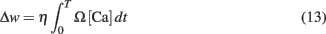where T = Nspike/f is the duration of the stimulation; Nspike is the number of spikes and f is the firing rate. The learning rate η = 0.01 was such that dww. The Ω function of calcium concentration [Ca] was a difference of two sigmoids,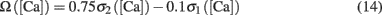where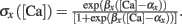Here we used β1 = 60, β2 = 100, α1 = 0.2, and α2 = 0.34. Basal levels of calcium concentration ([Ca] ≤ θd) produce no plasticity, while modest and high elevations of calcium generate LTD (θd ≤ [Ca] ≤ θp) and LTP ([Ca] ≥ θp) respectively. Notice that Ω reaches a maximal value for a given threshold of calcium θs ([Ca] ≥ 0.42 mM) above which the rate of LTP is constant. The function is shown in Figure 1A. For the rate-dependent simulations (Figures 15), pre- and postsynaptic stimuli were paired and delivered with presynaptic stimuli occurring 1 ms before the postsynaptic ones. Such paring was repeated 50 or 400 times at varying frequencies. Aperiodic stimuli were modeled as homogeneous Poisson process. Events occurring within less than 2 ms were discarded to incorporate refractoriness. For timing-dependent protocols, the time between pre- and postsynaptic spikes was varied between ±80 ms. All simulations, except the detailed neuron simulations (see below), were performed using Matlab. The differential equations were integrated using the fourth order Runge–Kutta method with a time resolution of 0.1 ms.

FIGURE 1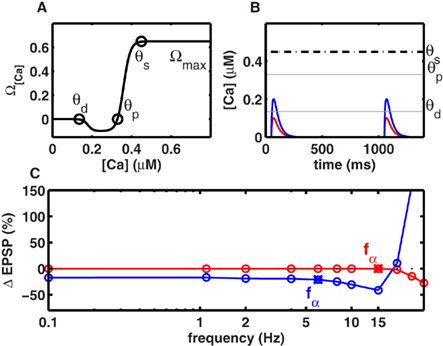Figure 1. Frequency-independent LTD at low rates. (A) The level of intracellular calcium concentration determined Ω[Ca], the direction and magnitude of synaptic modifications. Below the depression threshold θd (0.15 mM), no change in synaptic strength occurred. If calcium built up beyond θd, LTD was induced. For calcium levels above the potentiation threshold θp (0.33 mM), LTP was generated. Increasing calcium levels resulted in a stronger LTP until an upper limit θs (0.42 mM) was reached, beyond which synaptic changes occurred at a constant rate Ωmax (cf. Figure 3B). (B) Individual calcium transients during 2 Hz stimulation. Scenario I (red) when the NMDA-dependent calcium conductance gNMDA,Ca was small, single spike pairing did not lead to LTD. Scenario II (blue) when gNMDA,Ca was strong enough LTD was induced with single spike pairing. (C) Change in synaptic strength as a function of stimulation frequency. LTD was frequency-independent at frequencies below fα. The frequency fα was mainly determined by gNMDA,Ca, and the effective time constant of the system (τmax). In scenario I and scenario II (B) fα was 13 and 5 Hz respectively.

The model of plasticity described above can be considered as a hybrid model because it has both biophysical and phenomenological components. The biophysical components of the model are the dynamics of the EPSPs (Eqs 2–5), calcium currents (Eqs 6 and 9), and magnesium block (Eq. 7), which determines the strength of the calcium current. The phenomenological components of the model refer to the activity-dependent dynamics of the BPAP (Eqs 10–12), the stochasticity of synapses, and the estimation of the change in EPSP strength with calcium concentration (Eqs 13 and 14). We study the properties of this hybrid model of plasticity in both a point neuron model and a detailed model of CA1 neuron (see below).

### Detailed Neuron Model

The model of a CA1 pyramidal neuron was adapted from a previous study by Golding et al., 2001; http://senselab.med.yale.edu/ModelDB/ShowModel.asp?model = 64167). The multi- compartment model consisted of three active conductances: a voltage gated sodium conductance (gNa), a delayed rectifying potassium conductance (gKDR), and an A-type potassium conductance (gKA). The values of these conductances were taken from Migliore et al. (1999) and were inserted in all compartments of the cell, distributed as described in Figure 9 of Golding et al. (2001). Specifically, potassium conductance in the dendrites was set to 100 mS/cm2, independent of distance from the soma, while the sodium conductance increased linearly from 20 to 80 mS/cm2 as a function of distance from the soma. With this choice of active conductance in the dendrites, at 600 μm, the amplitude of an isolated BPAP decayed below 0.1 mV. In the absence of any external input, the neuron membrane time constant was 30 ms and the resting potential was set to −65 mV (for details see Golding et al., 2001). Synaptic currents were implemented as described by Eqs 2–4. In addition, calcium dynamics were modeled according to Eqs 6, 7, and 9. The detailed neuron simulations were performed using the NEURON simulation platform (Hines and Carnevale, 1997).

In the detailed model, we did not include the diffusion and redistribution of Ca++ in a synapse. Instead, we assumed that calcium is available for the processes leading to the induction of plasticity everywhere in the synapse. In other words, we modeled the synapse as a point, instead of having an extended volume. Based on the available data, we hypothesize that the results will not change qualitatively when a more detailed model of intra-synaptic Ca++ dynamics.

## Results

### Frequency-Independence of LTD

To understand the contribution of a non-saturating number of spikes to plasticity, we first investigated the case when spikes are delivered at low frequencies. Given the small time constant of the calcium transient (25 ms), at low stimulus frequencies (≤10 Hz) little, if any, temporal integration of calcium transients occurred across adjacent spikes in a stimulus. In this regime, we considered two scenarios. In Scenario I, individual calcium transients elicited by each spike were too small to cross the depression threshold θd (Figure 1B), resulting in no plasticity (Figure 1C). In general, repetitive stimulation at any frequency that was insufficient to promote temporal integration of calcium signals was unsuccessful in inducing LTD. In Scenario II, the NMDAR-mediated calcium conductance was higher, or the threshold θd for LTD induction was lower, so the calcium influx induced by individual spikes was sufficient to cross θd (Figure 1B). Again, for all frequencies where temporal integration was negligible, the duration for which calcium levels exceeded θd was identical, thereby generating identical amounts of LTD (Figure 1C). Both scenarios imply that below a critical frequency fα, the synaptic plasticity remains frequency-independent. Its magnitude is either identically zero (Scenario I, Figure 1C), or non-zero but constant (Scenario II, Figure 1C). Only when the stimulus exceeded a critical frequency fα did calcium integrate across adjacent spikes and produced LTD in a frequency-dependent manner. With the biologically realistic parameters, fα was 13 and 5 Hz in scenarios I and II respectively (Figure 1C).

Intuitively, fα is a critical frequency at which temporally adjacent calcium transients begin to summate, thereby altering the amount of LTD induced per spike. Thus, although fα can be influenced by many factors, it is mainly inversely proportional to the effective time course (τeff) of calcium transients. Conversely, the shape of experimentally measured LTD curves could be used to estimate τeff, the effective time course of calcium transients. For example, by pairing Schaffer collateral stimulation with CA1 whole-cell current injection at various frequencies, Johnston et al. (2003) observed significant frequency-independent LTD below 10 Hz. This corresponds to the Scenario II above, suggesting a τeff ∼ 50 ms, which is consistent with experimental observations of the calcium time course (Carter and Sabatini, 2004).

Further, the above simulations suggest that a change in the amplitude of the calcium transient will have a small effect on fα, but a substantial effect on the amount of LTD. Consistent with this prediction, Johnston et al. (2003) found that the addition of a small amount of NMDA antagonist, that presumably reduced the size of calcium transients, resulted in an absence of LTD below 10 Hz, and LTP above 30 Hz. Thus, the amount of LTD decreased significantly with the addition of NMDA antagonists, but the frequency at which LTD became frequency-independent remained largely unchanged. Although other mechanisms cannot be ruled out, these two experimental results can be explained by our model as arising from the underlying calcium dynamics.

### Preferred Frequency for Inducing Maximal LTP

At high frequencies, significant temporal integration occurred across adjacent spikes, which allowed calcium concentrations to cross the threshold θp for LTP induction. With the model assumptions stated above, we found a surprising frequency dependence of LTP: maximal LTP was induced at a frequency (fmax), while frequencies higher than fmax resulted in lesser LTP (Figure 2A). This can be understood as follows. At a certain frequency, temporal summation across adjacent spikes increased the effective size of calcium transients, which crossed the LTP threshold θp to induce LTP (Figure 2B). Increasing the stimulus frequency beyond this range produced larger calcium transients until eventually reaching the saturation level θs (Figures 2B and 1A). With the parameters used in this study (cf. Materials and Methods), calcium accumulation reached θs at ∼30 Hz. According to the third assumption (cf. Materials and Methods), all frequencies greater than 30 Hz were expected to induce the same amount of LTP per unit time, as determined by Ωmax. However, for a fixed number of spikes, an increase in stimulation frequency necessarily results in a decrease in stimulus duration (Figure 2B). Therefore, less LTP was induced at higher frequencies (Figures 2A,B) than at intermediate frequencies. This result can be derived analytically for frequencies above fmax. If Nspike is the number of spikes and f is the stimulation frequency, the stimulus duration is T = Nspike/f. If dw/dt is the rate of change of synaptic strength per unit time, the total change in synaptic strength Δw in a period T at frequencies greater than fmax is:

FIGURE 2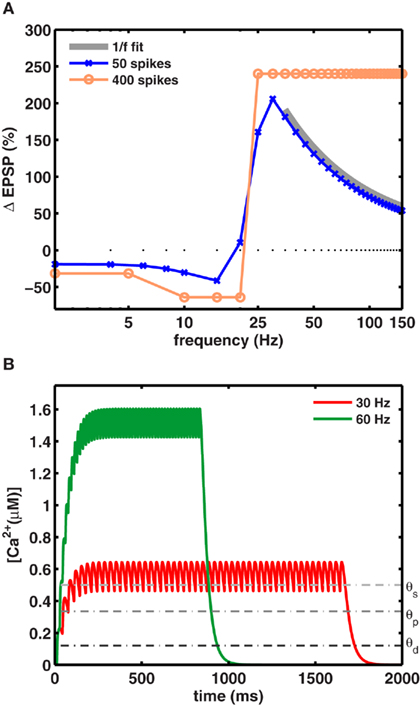Figure 2. A preferred frequency for inducing maximal LTP. (A) For a fixed number of spikes, maximal LTP was induced at intermediate frequencies fmax. The blue trace shows the dependence of synaptic weight change on the stimulation frequency (using 50 spikes). Maximal LTP (206%) occurred at fmax = 30 Hz for gNMDA,Ca = 1.23 θd (scenario II). The orange trace shows the plasticity induced by a large number of spike pairing (>400) which very likely saturated the synapses. With such a protocol the 1/f dependence was not observed and the relative change in synaptic strengths remained constant beyond fmax. The gray trace shows the 1/f dependence of LTP on stimulation frequency beyond fmax. (B) The increase in calcium concentration was higher for stimuli at 60 Hz (green), but its duration was half as long compared to the 30-Hz stimulation (red) for a fixed number of spikes. Calcium levels exceeded θs during stimulation in both cases to induce maximal LTP fmax per unit time.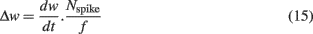For all frequencies above fmax, where calcium influx exceeds the critical value [Ca] ≥ θs, the plasticity per unit time is independent of calcium level and equal to the maximal value dw/dt = Ωmax (cf. Materials and Methods). Substituting this into Eq. 15above yields:Thus, fmax is the frequency that induces a maximal amount of plasticity per spike, such that at higher frequencies LTP decreases as 1/f. This dependence is illustrated in Figure 2A, where the LTP curve in the interval 35–150 Hz was fit with a function proportional to 1/f (thick gray line). Here, 30 Hz stimuli induced fourfold more plasticity than the same number of spikes applied at 150 Hz (Figure 2B).

The 1/f dependence of LTP is predicted to occur only when a small number spikes are used to induce plasticity. In fact, when plasticity is induced with a large number of spikes (>400), as is done in experiments which are designed to saturate LTP at all frequencies, the frequency dependence can be masked. This was tested explicitly in our model by using a saturating number of 400 spikes. As expected, in this case the model generated an equal amount of LTP at all high frequencies (Figure 2A pale blue trace), resulting in a plasticity curve that agrees with similar experimental data, and theoretical calculations. Notably, the 1/f dependence of LTP would be unaltered if the synapses did not saturate. That is, for a small number of spikes that typically occur during behavior and are unlikely to saturate synapses, the model makes a novel and testable prediction about the rate-dependence of LTP. It also suggests that among other parameters, at frequencies above ∼30 Hz, the number of spikes in the stimulus is itself a crucial parameter in determining the amount of plasticity.

### The Preferred Frequency fmax for Inducing LTP Depends on Calcium Dynamics

In Figures 1 and 2 we used a calcium time course of 25 ms. However, experimentally measured values of the calcium time course take a wide range of values depending on the brain region and neuron type (Helmchen et al., 1996; Carter and Sabatini, 2004; Yuste and Konnerth, 2005). To understand the effect of the calcium time constant we systematically varied its value from 25 to 200 ms while keeping the total amount of calcium per spike constant. We found that within a biologically realistic range of values of the calcium time constant, the value of fmax, and the 1/f dependence of LTP, remained effectively unchanged (Figure 3A). The calcium time course will have a significant impact on the frequency dependence of LTP when the duration of calcium far exceeds the duration of stimulation.

FIGURE 3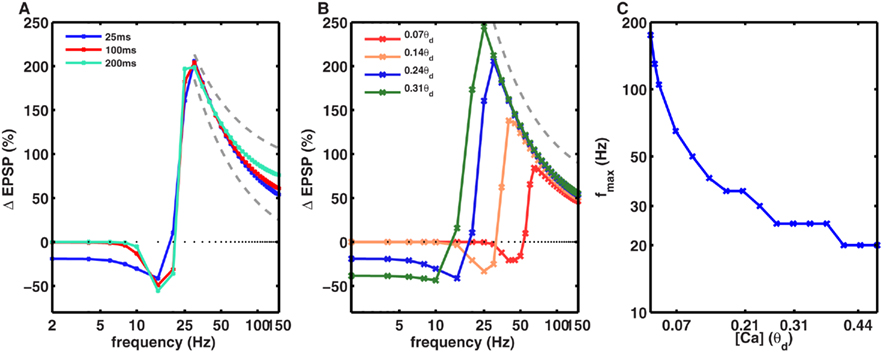Figure 3. Dependence of frequency fmax for inducing maximal LTP on the amplitude and duration of calcium transients. (A) The time course of calcium transient τCa was varied from 25 to 200 ms (solid lines) while keeping the area under the curve for the calcium transients constant. With 50 spikes, the frequency fmax was identical for all values of τCa. Similarly, the 1/f dependence of LTP (gray dotted line) beyond fmax was also unaltered by changes in τCa (dotted gray line). (B) In contrast, the amplitude of calcium transients, gNMDA,Ca, affected the fmax such that an increase in gNMDA,Ca reduced fmax. Note that here the amplitude of the Ca transient is shown in units of θd. The 1/f dependence of LTP beyond fmax was unaffected by the amplitude of gNMDA,Ca. (C) Dependence of fmax on gNMDA,Ca. For very low calcium permeability the fmax was as high as ∼200 Hz, whereas for high values of gNMDA,Ca, fmax was close to 10 Hz. Note that the y-axis is on a log-scale.

Next, we investigated the effect of varying the amplitude of the calcium transients, which was measured relative to the model parameter θd. To isolate the effect of amplitude, we fixed the temporal extent of the calcium transients and varied their size. We found that increasing the amplitude of the calcium transients reduced the fmax (Figures 3B,C). In other words, synapses with higher calcium permeability would need lower frequency stimulation to induce maximal LTP. Notably, even though fmax varied from 20 to ∼200 Hz, the 1/f dependence of LTP was observed for all the values of fmax (Figures 3B,C).

Consistently, robust LTP has been induced in vitro at rates ranging from 40 to 400 Hz (Trepel and Racine, 2000; Johnston et al., 2003). However, a direct numerical comparison between the model and experiments is difficult because the model calculates the rate-dependence of an isolated NMDA-dependent synapse, whereas typical in vitro experiments stimulate a large number of synapses that could cooperate and change the preferred frequency for LTP. Further, the presence of inhibition and non-NMDA-dependent mechanisms such as voltage gated calcium channels may also influence the rate-dependence of LTP. Nonetheless, the novel predicted rate-dependence of an NMDA-dependent synapse would contribute to plasticity induction in complex networks.

### BPAP Amplitude Can Induce a Switch in the Direction of Plasticity

The BPAP amplitude attenuates as a function of both spike frequency and distance from the soma. While BPAP can be 100 mV at proximal dendrites, it may decay completely by the time it reaches a distal synapse (Golding et al., 2001). The BPAP amplitude attenuation as a function of spike frequency is small near the soma (about 20%), and was incorporated in the above simulations. To understand the influence of these short-term BPAP dynamics on synaptic plasticity, we simulated a multi-compartment model CA1 pyramidal neuron (cf. Materials and Methods). The BPAP amplitude showed dendritic-position and stimulation frequency-dependent attenuation such that at 0.1 Hz stimulation the BPAP amplitude was insignificant (∼0.1 mV) beyond 500 μm from the soma (Spruston et al., 1995; Golding et al., 2001). This multi-compartment, biophysical neuron also showed the rate-dependence of LTP and LTD (Figure 4A). The value of fmax producing maximal LTP was larger for synapses located farther from the soma (Figure 4A). The change in fmax with diminishing BPAP amplitude was also captured by the reduced model (Figures 1A and 4B).

FIGURE 4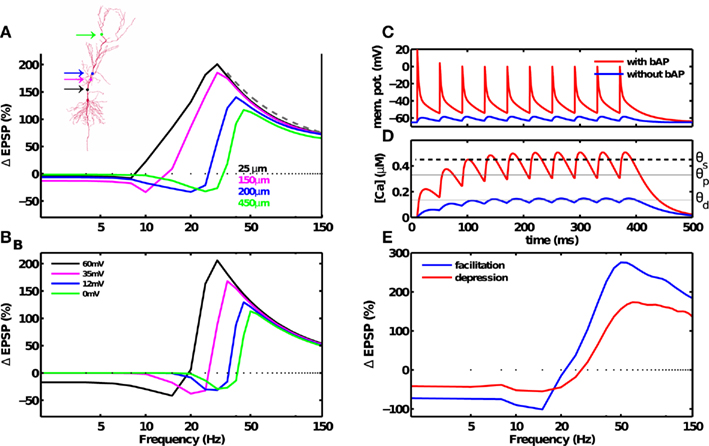Figure 4. Dendritic and synaptic short-term dynamics influences the frequency fmax for inducing maximal LTP, but not the 1/f dependence of LTP. (A) Morphology of a CA1 pyramidal neuron. Simulations were carried out with a multi-compartment, biophysical model of a CA1 pyramidal neuron (inset). The dendritic Na+ and K+ conductance were adjusted such that BPAP amplitude decayed with distance from the soma (cf. Materials and Methods). BPAP amplitude also decayed with repeated stimulation. At a distal synapse (green dot) the BPAP amplitude was insignificant (∼0.1 mV). Synaptic plasticity induced by a fixed number of spikes at various frequencies was dependent on the location of the synapse on the dendritic arbor. As the synapses’ distance from the soma was increased (solid lines, colors correspond to the synapse position shown in the inset), from the most proximal synapse (25 μm) to the most distal synapse (450 μm), the maximal amount of LTP decreased from 188 to 108%, and the frequency fmax for inducing maximal LTP increased from 30 to 45 Hz. The 1/f dependence of LTP beyond fmax was unchanged. (B) Similar results were obtained using a reduced, single-compartment model with a range of BPAP amplitudes (colored lines). (C) Membrane potential at the location of the synapse in the postsynaptic neuron in the presence of a strong BPAP (red trace), or without the BPAP (blue trace). (D) Calcium concentration at the synapse in the postsynaptic neuron in the presence of a strong BPAP (red trace), or without the BPAP (blue trace). (E) The frequency dependence of LTD and LTP remains unaffected by frequency-dependent depression (red). Adding frequency-dependent synaptic facilitation (blue) increased the amount of plasticity compared to a short-term depressing synapse. Notably, neither depression or facilitation altered the 1/f dependence of LTP beyond fmax.

Notably, LTP was induced at even the most distal synapse simulated (500 μm, fmax ≈ 50 Hz) where the BPAP amplitude was very small. Here, temporal summation of adjacent EPSPs generated sufficient depolarization to remove the magnesium block, and induce large calcium influx and plasticity (Figures 4C,D). The dendritic distance-dependent reduction of the BPAP amplitude was thus responsible for the increase in fmax at distal synapses. By the same token, an intermediate frequency (e.g., 20 Hz) that induced LTP at a proximal synapse (Figures 4A,B, black trace) induced LTD at a distal synapse (Figures 4A,B, blue or green trace). Such a dendritic-position dependent switch from LTP to LTD has already been observed experimentally (Letzkus et al., 2006; Sjöström and Häusser, 2006).

Here, we excluded the effect of boosting of the BPAP by EPSP-induced depolarization of the dendrite (Waters and Helmchen, 2004) for two reasons. First, this effect is pronounced only at the distal dendrites whereas our key results hold even at the proximal dendrites. Second, the BPAP amplitude in distal dendrites decreases as a function of the dendritic distance, as well as with repeated activation. While dendritic depolarization boosts the amplitude of individual BPAPs, the effect of dendritic depolarization on repeated, high-frequency activation of BPAPs is unknown and our model investigates precisely this parameter regime. As long as the depolarization-induced boosting of BPAP keeps the BPAP amplitude in the distal dendrites smaller than the BPAP in the proximal dendrites, which is shown by most experiments, our results would remain qualitatively unchanged.

### Short-Term Dynamics Do not Abolish the Rate-Dependence of LTP

In addition to receiving BPAP-induced depolarization, synapses also show short-term dynamics, such as frequency-dependent facilitation and depression (Markram et al., 1997; Klyachko and Stevens, 2006). To investigate the effects of short-term synaptic dynamics on LTP, it is important to consider the stochastic nature of synaptic transmission. The transmission probability P was assumed to be dependent on the frequency of presynaptic spikes (cf. Materials and Methods). As expected, frequency-dependent facilitation resulted in more potentiation than depression. Notably, the 1/f dependence of LTP on frequency was unchanged when considering frequency-dependent stochastic synapses (Figures 4E and 6D,F). These results demonstrate that while short-term dynamics of the BPAP and synapses changed the preferred frequency of LTP induction, they did not abolish the predicted rate-dependence of LTP.

### Enhanced Plasticity with Periodic Stimuli

The above simulations used periodic stimuli, which are predominantly used in in vitro experiments. Similarly, natural spike trains often display rhythmic activity (Buzsaki, 2006), which is thought to be critical for learning and memory (Winson, 1978). By contrast, neurons also fire in an irregular, aperiodic fashion, where the spike trains can be approximately described by Poisson statistics. To understand the role of rhythmic activity in learning, we compared the amount of plasticity induced by Poisson-distributed and periodic spike trains with equal mean firing rates and numbers of spikes.

We simulated a synapse receiving 50 spikes obeying homogeneous Poisson statistics with different average firing rates. The resulting plasticity curve showed significantly reduced magnitudes of LTD and LTP compared to the plasticity generated by periodic stimuli (Figures 5A and 6E,F). The peak-to-peak amplitude of the rate-dependent modulation of plasticity (max. LTP – max. LTD) also decreased from ∼240% in the periodic case, to ∼140% in the Poisson case. The peak LTP was reduced to 130% with Poisson type stimuli because even high average-rate stimuli contain some instances of low rate spiking (Figures 5B,C). The peak LTD was reduced dramatically (∼10%) with Poisson type stimuli. This decrease in the amount of LTD can be attributed to the presence of bursts of spikes with high intra-burst rates occurring in a low rate Poisson spike train (Figures 5B,C). These results indicate that periodic stimuli dramatically and significantly enhance LTP and LTD compared to irregular stimuli. This provides a mechanism, at the single synapse level, for the facilitating role of neural oscillations in learning and memory (Winson, 1978).

FIGURE 5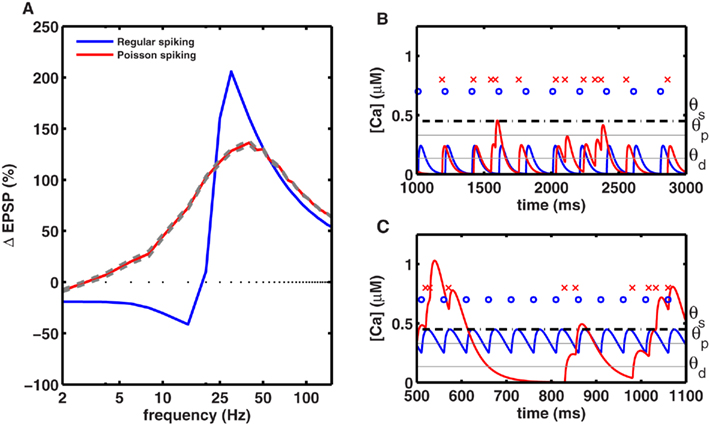Figure 5. Periodic stimuli induce stronger plasticity than Poisson-distributed stimuli. (A) Plasticity was induced with 50 spikes, delivered at various frequencies in either a periodic fashion (blue) or in a Poisson-distributed fashion (red), using the same mean rate. The amount of plasticity (averaged across 20 trials) induced by homogeneous Poisson spike trains, with mean rates indicated on the x-axis, is shown in red (with gray lines indicating the SE). The amplitude of the plasticity curve is smaller with Poisson stimuli (maximal LTP = 137 ± 1.8% at 40 Hz, maximal LTD = 10 ± 0.4% at 2 Hz) than with periodic stimulation (maximal LTP = 206% at 30 Hz, maximal LTD = 41% at 15 Hz). (B) Comparison of the calcium time course with periodic and aperiodic (Poisson type) stimuli delivered at a low rate. Top row indicates periodic spikes (blue dots) and aperiodic spikes (red crosses). The corresponding calcium transients for periodic (blue line) and aperiodic (red lines) inputs are shown at the bottom. The low rate aperiodic stimuli occasionally contain bursts of spikes that cross the threshold for inducing LTP, while they induce LTD at other times. Due to this interference, the low rate aperiodic stimuli induce less LTD than periodic ones. (C) Same as in (B) but for high-rate stimuli. Once again, aperiodic stimuli fluctuate between high and low rates resulting in both LTD and LTP, such that the net LTP is less with aperiodic stimuli than with periodic ones.

FIGURE 6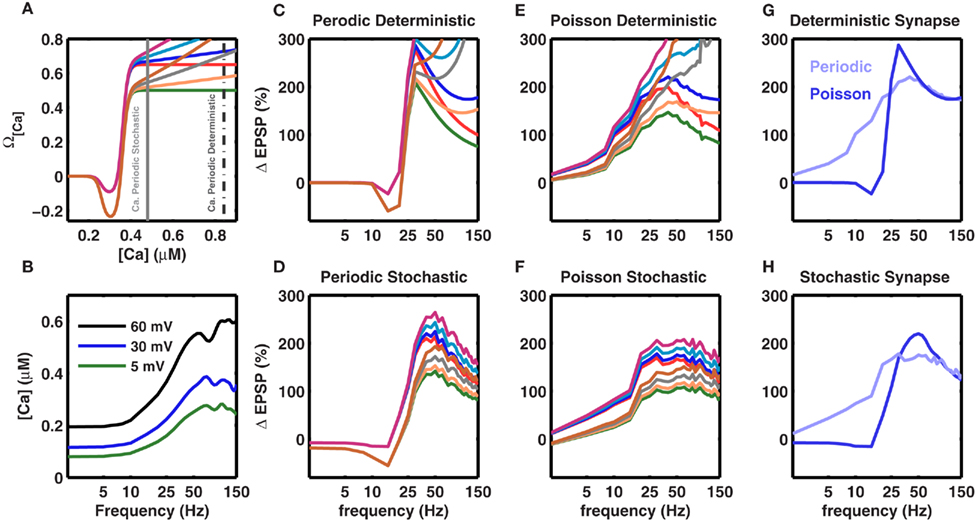Figure 6. Robustness of the frequency fmax for inducing maximal LTP to changes in the model parameters. Experiments show that the amount of LTP is a saturating function of the amplitude of the calcium transient. This is modeled by the Ω function (cf. Figure 1A main text), which saturates at high values and makes [Ca] (and the amount of LTP) frequency-independent at high frequencies. Here we tested the effect of different non-saturating Ω functions (A) Different Ω functions with either saturating or non-saturating behavior at high calcium concentrations. The two vertical gray lines mark the calcium concentration for periodic (broken gray) and Poisson type spike trains (solid gray) at 50 Hz. (C–F) Effect of a non-saturating Ω function on the frequency dependence of LTP beyond fmax. The colors of the traces are matched with the corresponding Ω function shown in (A). (B) Calcium concentration in a stochastic synapse for three different BPAP amplitudes as a function of stimulation frequency. Each trace shows the average of 50 trials. At higher frequencies the amount of calcium in a spine tends to saturate or even decrease for distal synapses due to dominating frequency-dependent depression. (C) Frequency dependence of LTP for a periodic (regular) spike train impinging on a deterministic synapse. (D) Frequency dependence of LTP for a periodic spike train impinging on a frequency-dependent and stochastic synapse. (E) Frequency dependence of LTP for a Poisson type spike train impinging on a deterministic synapse. (F) Frequency dependence of LTP for a Poisson type spike train impinging on a frequency-dependent and stochastic synapse. Evidently, with deterministic synapses, independent of the spike pattern, the 1/f dependence of LTP is not observed even for small changes in the saturation of the Ω function. By contrast, with stochastic synapses saturation of the Ω function is not required to observe the 1/f dependence of LTP beyond fmax because the amount calcium saturates (B). Thus, the 1/f behavior of LTP is robust for stochastic synapses. (G) Comparison of frequency-dependent plasticity induced by either periodic (blue) or Poisson type spike trains (pale blue), when the synapse was modeled as deterministic. The blue trace is the same as in (C), while the pale blue curve is plotted as the blue trace in (E). (H) Same as in (G) for the stochastic synapse model. (G,H) Show that independent of the synapse model, periodic spike trains induce more plasticity than Poisson type spike trains. However, note that at high frequencies, with the stochastic synapse model, both periodic and Poisson type spike trains induce the same amount of plasticity. In (C–H), the traces represent an average of 50 trials.

### Effect of a Non-Saturating Ω Function on the Rate-Dependent Plasticity

The above calculations used the experimentally observed, saturating dependence of LTP on calcium concentration (Figure 1A). Therefore, it is important to understand to what extent these results depend on the assumption that the Ω function saturates at high values of calcium. Hence, we relaxed this constraint and considered several variations of a non-saturating Ω function such that LTP was allowed to increase indefinitely as a function of calcium levels (Figure 6A). With this modification in the Ω function, i.e., without any saturation at high values, the frequencies fα and fmax remained unaltered (Figure 6). The 1/f dependence was more affected when using deterministic synapses (Figures 6C,E). In contrast, stochastic synapses continued to exhibit approximately 1/f LTP (Figures 6D,F). This is because the calcium concentration saturates at high frequencies due to the frequency-dependent depression in stochastic synapses (Figure 6B). Thus, a saturating Ω function was not required to obtain a frequency-dependent decrease in LTP beyond fmax, and the novel rate-dependence of LTP was robust, surviving relaxations of many assumptions of the model as well as large and dynamic changes in synaptic strengths and the BPAP amplitude.

### Joint Influence of Spike Timing and Spike Rate on Plasticity

In addition to spike rate, synaptic plasticity critically depends on spike timing (Magee and Johnston, 1997; Markram et al., 1997; Nishiyama et al., 2000; Bi and Poo, 2001; Sjöström et al., 2001; Wittenberg and Wang, 2006). The postsynaptic calcium influx is a determining factor in STDP induction (Magee and Johnston, 1997), and STDP has been observed in several brain regions including the neocortex (Markram et al., 1997; Sjöström et al., 2001), hippocampal cultures (Bi and Poo, 1998, 2001), and in hippocampus in vitro (Nishiyama et al., 2000; Wittenberg and Wang, 2006). Consistent with these hippocampal in vitro experiments (Nishiyama et al., 2000; Wittenberg and Wang, 2006), our model showed that when delivered at 5 Hz, pairs of pre- and postsynaptic spikes induced LTD at all pre-post spike latencies between ±50 ms (Figure 7A).

FIGURE 7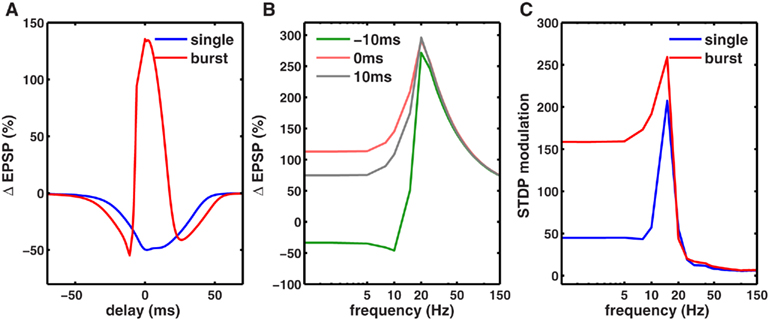Figure 7. Co-dependence of synaptic plasticity on spike rate and timing. (A) Each presynaptic spike was paired with a postsynaptic spike at 0.1 Hz, and the latency between the pre-post spike-times was varied between (−80 and +80 ms. The synaptic strength gNMDA,Ca was chosen to be in scenario II (Figure 1B, Materials and Methods). Here, for all latencies between ±50 ms LTD was induced. However when each presynaptic spike was paired with a burst of two postsynaptic spikes (inter spike interval ISI = 10 ms), LTP was induced between −5 and +10 ms. LTD was induced in two temporal windows: between −40 and −5 ms and between +10 and +60 ms. (B) Rate-dependence of synaptic plasticity for three different values of spike timings or latencies between pre- and postsynaptic spikes (red = 0 ms, gray = 10 ms, green = −10 ms). Here, each presynaptic spike was paired with two postsynaptic spikes (ISI = 10 ms). Spike timing had a strong timing effect on the amount of plasticity induced at low frequencies. However, at rates greater than fmax, spike timing had no impact on synaptic plasticity. (C) The modulation of synaptic plasticity by spike timing was computed as the difference between maximal and minimal plasticity (see text) for all latencies at a given rate. The timing dependence of plasticity was maximal in a narrow range of rates just below fmax (blue). Pairing each presynaptic spike with a burst of two postsynaptic spikes widened the range of frequencies (red) where timing dependence was significant.

Spike bursts strongly influence STDP (Sjöström et al., 2001; Wittenberg and Wang, 2006). Consistently, we found that robust LTP was induced when a presynaptic spike was paired with a 100-Hz burst of two postsynaptic spikes within −5 and +15 ms, whereas LTD was induced at all other latencies up to ±50 ms (Figure 7A; Nishiyama et al., 2000; Sjöström et al., 2001; Wittenberg and Wang, 2006). Here, the synapses were in scenario II (Figure 1B), where at a low rate calcium transients induced by pairing one pre- and one postsynaptic spike were too small to induce LTP, but were large enough to induce LTD. Increasing the latency between pre- and postsynaptic spikes reduced the amplitude of the calcium transient and hence the amount of LTD. Pairing a presynaptic spike with a burst of postsynaptic spikes nearly doubled the size of the calcium transient, which was large enough to cross the LTP threshold at small latencies. Similar results would be obtained if the size of the calcium transient were made larger, resulting in LTP at short latencies (Sjöström et al., 2001). Thus, the model can capture the joint influence of spike timing and postsynaptic bursting on STDP.

We next investigated the joint influence of spike rate and timing on plasticity (Figure 7B). At rates higher than the frequency fmax for maximal LTP induction, there was no timing dependence of plasticity because the adjacent spikes overlapped at all latencies, generating identically large calcium influx and LTP. At low frequencies, adjacent spikes were far enough apart such that the calcium influx could be modulated by spike timing (Figure 7B). In agreement with this, timing-dependent plasticity has been observed experimentally at low rates (Sjöström et al., 2001).

To determine the influence of spike timing on plasticity induced at each rate, we computed the difference between maximal LTP and minimal LTD induced at any latency for each rate (Figure 7C), termed STDP modulation. Interestingly, maximal STDP modulation was observed at a rate slightly less than the optimal frequency fmax. At rates much lower than fmax the STDP modulation was low, while at rates higher than fmax there was no significant STDP modulation. Thus, STDP modulation by spike rate was restricted to a narrow range (∼5-15 Hz) when only one postsynaptic spike was paired with a presynaptic spike. However, postsynaptic bursting significantly increased the range of frequencies where strong STDP modulation occurred. Similar results were obtained for stochastic synapses (Figure 8). These results show a novel interaction between spike timing, spike rate, and spike bursting, that determines the direction and the magnitude of plasticity.

FIGURE 8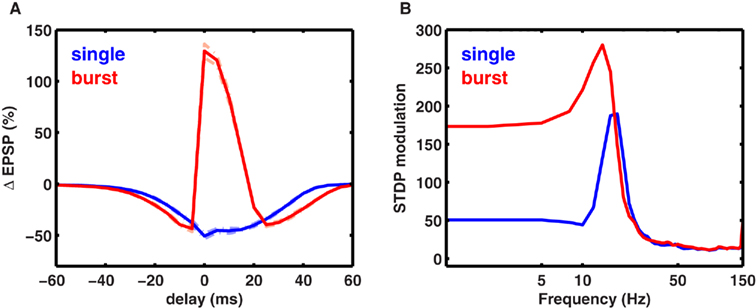Figure 8. Spike timing-dependent plasticity with stochastic synapses. (A) Average change in EPSP amplitude for a stochastic synapse (cf. Materials and Methods) when one presynaptic spike is paired with one postsynaptic spike (blue) or with two postsynaptic spikes (red). The dotted lines show the SD for the two traces. (B) Modulation of synaptic plasticity for stochastic synapses. Similar to the deterministic synapses, the rate- and timing-dependent plasticity work together to give maximal modulation between ∼5 and 20 Hz.

## Discussion

Relative to standard plasticity induction protocols, natural spike trains contain a small number of spikes with variable spike rate and rhythmicity. Moreover, the latency between the pre- and postsynaptic spikes, as well as the number of spikes generated in a postsynaptic neuron in response to one presynaptic spike can vary. Here, we computed the influence of these variables on the nature of synaptic plasticity using a biophysical model of an isolated NMDA-dependent synapse (Artola and Singer, 1993; Karmarkar and Buonomano, 2002; Shouval et al., 2002; Abarbanel et al., 2003; Cai et al., 2007). The model could explain a vast amount of data on both the rate and timing dependence of synaptic plasticity. Thus, the model provides a unified theoretical explanation of those experimental observations, and also makes novel predictions. The predictions of the simple, reduced model that used deterministic synapses were confirmed using more biophysical models that used stochastic synapses, short-term dynamics of EPSPs and BPAP, and a multi-compartment neuron incorporating the activity-dependent dynamics of dendrites.

### Model Predictions and Their Significance

(1) A key finding was that for a fixed number of spikes used for inducing plasticity, as is commonly done in most experiments, maximal LTP was induced at a frequency fmax (Figures 2 and 3). For spikes delivered at frequencies higher than fmax the amount of LTP induced decreased as ∼1/f. That is, with biologically realistic parameters, at frequencies above ∼30 Hz, the number of spikes is a crucial parameters that determines the amount of plasticity of a synapse and the amount of LTP induced per spike reduced with increasing stimulation frequency. Alternatively, the number of spikes and the stimulus duration are key parameters when synapses are paired with tens of spikes. This effect disappears when hundreds of spikes are used, that saturate plasticity. Hints of the inverted-U frequency dependence can be found in some experimental studies (Berry et al., 1989; Wang and Wagner, 1999). However, most studies do not report such frequency dependence, potentially due to two reasons: (1) few studies have probed the sufficiently high-frequency regime; (2) most studies use a large number of spikes to induce LTP or LTD which saturates synaptic strengths, thereby potentially masking the novel frequency dependence in the sub-saturating regime. Indeed, in the saturating regime, the plasticity curve predicted here converges to the standard shape with a sufficiently large number of stimulus spikes (Figure 2 pale blue trace). In order to test the novel predictions of the model, an experiment is needed that uses a small number of spikes to induce plasticity without saturating the synaptic strengths. Further, the experiment would need to span a wide range of frequencies to detect a decrease in LTP with higher frequency stimulation, and test the predicted 1/f dependence of LTP at an NMDA-dependent synapse.

The predicted 1/f frequency dependence of LTP was robust to a number of model parameters and assumption, including short-term plasticity (Figures 4E and 6D,F), the dendritic location of the synapse (Figures 4A,B) and spike statistics (Figure 5A). Synaptic facilitation lowered the value of fmax where maximal LTP occurred, and synaptic depression increased fmax, but the 1/f dependence of LTP beyond fmax was unaltered in all cases. Similarly, the dendritic distance, and stimulus frequency-dependent attenuation of BPAP changed the numerical value of fmax but did not influence the 1/f dependence of LTP (Figures 4A,B). Notably, the 1/f dependence of LTP persisted even at the distal synapses where the BPAPs were maximally attenuated. In such cases, LTP was induced entirely by cooperation between temporally adjacent EPSPs, with no significant contribution from BPAPs. These results were confirmed using a detailed, multi-compartment model of a CA1 neuron. Thus, the novel 1/f dependence of LTP on stimulation frequency is a robust phenomenon, and persists with strong short-term plasticity. This prediction can be tested experimentally by inducing LTP at an isolated synapse with pharmacological manipulations that alter the amount of synaptic facilitation and depression. Observation of a 1/f dependence of LTP with different levels of synaptic facilitation and depression would validate the model.

(2) In our model, rhythmic stimuli generated far greater LTP and LTD than arrhythmic (Poisson type spike trains) stimuli (Figure 5A). Several experiments have shown that neural activity becomes periodic under learning and memory tasks, and that abolishing these oscillations results in learning deficits (Winson, 1978; Stopfer et al., 1997; Fries et al., 2001; Buzsaki, 2006). On the other hand, enhancement of neural oscillations at specific frequencies can boost memory (Marshall et al., 2006). Our model provides computational supports for these results and suggests that oscillations may play an important role in learning and memory by facilitating LTP and LTD in a frequency-dependent fashion at the level of a single synapse. This prediction is easily testable experimentally. Observation of a greater amount of plasticity at any stimulation rate with periodic stimuli compared to aperiodic ones would provide a strong support for our model, and suggest that oscillations can play a role in learning at the level of an individual synapse.

(3) We investigated the joint effects of variation in spike timing and spike rate on the resulting plasticity. We found that the modulation of plasticity by spike timing was maximal at stimulation rates just below fmax. Stimuli with rates higher than fmax showed plasticity with virtually no timing dependence. Further, the timing dependence of plasticity was restricted to a narrow window (∼5-15 Hz) when each presynaptic spike was paired with one postsynaptic spike (Figures 7B,C), but less so when presynaptic spikes were paired with bursts of postsynaptic spikes. This finding can be tested experimentally by measuring the timing dependence of LTP with or without postsynaptic spike bursts. Stronger amount of STDP with spike bursts than with single spikes would validate our model.

The sharp change in the timing sensitivity of plasticity as a function of spike rate could have a profound influence on network models of learning. Further, we found that pairing each presynaptic spike with a burst of postsynaptic spikes dramatically increased the range of firing rates where spike timing exerted a significant effect on synaptic plasticity. Thus, state-dependent changes in neural activity from single spike to burst mode could have a strong impact on the resulting plasticity and learning. In single spike mode, networks would be more likely to learn based on overall correlations, such as through a rate code, whereas during the burst mode networks would be more likely to learn based on precise spike timings, or through a temporal code.

(4) Various physiological parameters can alter the frequency fmax at which maximal LTP is induced. For example, the amplitude of the BPAP is reduced with increasing dendritic distance from the soma (Spruston et al., 1995; Magee and Johnston, 1997). Thus, greater cooperation between adjacent spikes is required at distal synapses to cross the LTP threshold, resulting in an increase of fmax. Thus, if maximal LTP is induced at a low frequency, e.g., 10 Hz, at a proximal synapse, maximal LTP would be induced at a higher frequency, e.g., 30 Hz, at an identical distal synapse. Further, the result that low frequency stimuli could induce LTP at a proximal synapse while inducing LTD at a corresponding distal synapses is consistent with experimental findings (Sjöström and Häusser, 2006). Thus, the plasticity rule predicted here could use the same input rate to differentially modulate the direction and magnitude of synaptic plasticity depending on their dendritic location. We speculate that the dendritic location-dependence of preferred frequency for LTP induction may have strong implications for the influence of attention on learning. Typically, the bottom-up inputs to the neocortex arrive at proximal dendrites, whereas top-down inputs from higher cortical areas, which putatively carry information related to attention, arrive at distal dendrites.

Our model predicts that the preferred frequency for inducing LTP is higher at the distal dendrites compared to the proximal ones (Figures 4A,B). Hence we speculate that higher frequency oscillations may facilitate top-down learning of inputs arriving at distal synapses, whereas lower frequency oscillations may facilitate learning of the bottom-up inputs at proximal dendrites (Buschman and Miller, 2007). In addition to the network based mechanisms (Niebur et al., 1993; Sivan and Kopell, 2004), this could also provide another single synapse based mechanism by which the high-frequency gamma oscillations, that typically appear in tasks involving attention, can facilitate learning (Winson, 1978; Fries et al., 2001; Buzsaki, 2006). Further, our results can explain recent findings showing that glutamatergic inputs from the entorhinal cortex and area CA3, terminating on the distal and proximal dendritic segments of area CA1, prefer faster- and slower-frequency gamma oscillations respectively during spatial exploration (Colgin et al., 2009; Chen et al., 2011). Our results suggest that the fast and slow gamma oscillations are best suited for inducing maximal plasticity for the entorhinal and CA3 inputs on CA1 respectively.

In addition to the BPAP amplitude, the calcium permeability in the spines also affected fmax without altering the 1/f dependence. For very low-permeability synapses, fmax was as high as 200 Hz. In vivo LTP induction protocols in rat neocortex have reported induction of LTP with eight spikes at 300 Hz (McNaughton et al., 1978; Trepel and Racine, 2000). Our model suggests that synapses with low calcium permeability may be responsible.

(5) Our model predicts that below a certain frequency fα, LTD is independent of frequency (Figure 1C). The value of fα is inversely proportional to the time course of the stimulus-evoked calcium transient. Thus, the shape of the LTD curve, which can be measured in vivo, could provide an estimate of the time course of intracellular calcium transients, without explicit knowledge of calcium buffering kinetics. This is useful because direct measurements of the calcium time course are difficult due to the interference caused by calcium indicators (Helmchen et al., 1996; Carter and Sabatini, 2004; Yuste and Konnerth, 2005). Moreover, exact estimates of calcium dynamics also require knowledge of calcium buffering kinetics, which is even more difficult to measure. Preliminary evidence for the frequency-independence of LTD predicted by our model can be found in available experimental data (Wang and Wagner, 1999; Johnston et al., 2003).

These results are based on a few commonly held and experimentally supported assumptions, which have been successful in explaining saturated state synaptic plasticity. The novel findings of an optimal frequency for inducing LTP and enhanced plasticity with periodic stimuli compared to aperiodic ones are generally insensitive to model parameters. There is only one parameter that largely determines this behavior, namely θs, the value of calcium concentration beyond which an increase in calcium levels does not lead to increased LTP. Change in θs would alter the preferred frequency for inducing maximal LTP, but the results would remain qualitatively unchanged. In fact, not only the model parameters, but also some of the assumptions of the model can be changed without a qualitative change in the results. For example, it is possible that the plasticity rule itself has a calcium dependence in the sub-saturated regime that differs from the one measured in the saturated state. In particular, it is conceivable that the rate of LTP induction does not plateau above θs, but continues to increase with increasing levels of calcium. It can be easily deduced from our model (Figure 6) that, as long as such growth is sub-linear, the decline of LTP at high frequencies will be maintained, although it may not take the characteristic 1/f dependence. In addition, the stochastic and frequency-dependent behavior of a synapse can restore the 1/f dependence of LTP beyond fmax, even with a completely non-saturating Ω function (Figure 6).

We have assumed that plasticity occurs incrementally with repeated stimulation. In this regard, it has been suggested that single synapses operate as devices with two or more discrete states, such that plasticity occurs through switch-like transitions between these states (Petersen et al., 1998; O’Connor et al., 2005). However, neurons typically make multiple contacts on their postsynaptic targets, each with a different threshold for plasticity induction. The commonly observed graded plasticity could result from switching different fractions of the synapse population. In this scenario, our analysis would describe the collective response of an ensemble of synapses with a continuous range of plasticity thresholds. It has been suggested that a small number of stimuli fail to induce long-term synaptic plasticity at CA3–CA1 synapses, let alone saturating LTP (Fink and O’Dell, 2009). On the other hand, recent experiments involving precisely timed stimulation of a pair of pre- and postsynaptic neurons show incremental change in synaptic strength with increasing number of stimuli (Zhang et al., 2009). More experiments are needed to determine if physiologically realistic, small numbers of spikes can induce LTP, and how it depends on stimulus parameters.

Further, other factors, such as metabotropic glutamate receptors, can have complex effects on the NMDA-dependent plasticity (Nevian and Sakmann, 2006). Such effects could be incorporated by expanding the model to include a more detailed model of the presynaptic neuron (or bouton) and extracellular signaling pathways. For simplicity, we have considered only the contribution of postsynaptic NMDAR-mediated influx of plasticity-inducing calcium ions. Thus, here we have only focused on postsynaptic mechanisms of synaptic plasticity. In our current model we have also excluded additional sources of calcium, such as voltage gated calcium channels and intracellular calcium stores. However, addition of such mechanisms would not change our predictions in a qualitative manner if the following conditions are met: First, synaptic modifications depend on both the amplitude and the duration of a given plasticity-inducing signal. Second, the amplitude of the signal determining the synaptic modification integrates with repeated stimuli, with the caveat that the amount of plasticity it produces is limited from above.

The model does not take in to account the influence of inhibition during natural behavior. In the simple case that spikes are periodic and excitation and inhibition occur at opposite phases of the oscillation, the major findings are likely to remain unaltered. However, precisely timed inhibition could alter the magnitude of the BPAP, and thus could influence the fmax. More experimental and theoretical studies are required to understand the interaction between excitatory and inhibitory spike timing and synaptic plasticity. Similarly, more experimental and theoretical studies are needed to understand how the novel plasticity rule predicted here will influence the connectivity and dynamics of a network of excitatory neurons.

### Relationship with Other Models

Over the last decade a number of reduced phenomenological (Guetig et al., 2003; Pfister and Gerstner, 2006), hybrid (i.e., phenomenological and biophysical; Karmarkar and Buonomano, 2002; Shouval et al., 2002, 2010; Abarbanel et al., 2003; Cai et al., 2007; Clopath et al., 2010), and biologically realistic models (Graupner and Brunel, 2010) have been proposed to explain one or more aspects of STDP. In the reduced model, the main aim is to find a mathematical function that can fit the experimentally observed STDP curves (Bi and Poo, 1998). In the hybrid models of synaptic plasticity, the membrane potential and calcium dynamics are explicitly modeled using Hodgkin and Huxley type equations, while the amount of plasticity is assumed to be proportional to the calcium influx in a spine. More detailed biophysical models have been used to relate the biochemical reactions responsible for changes in calcium concentration to the resulting induction and maintenance of plasticity (Graupner and Brunel, 2010). Hybrid models have provided important clues about the influence of various key parameters, such as the EPSP time constant, the magnitude and time course of calcium transients, and the shape of the BPAP, on the amount of plasticity (Karmarkar and Buonomano, 2002; Shouval et al., 2002; Abarbanel et al., 2003; Cai et al., 2007).

On the other hand, the reduced and the phenomenological models, which are more suitable for large network simulations, have furthered our understanding of how STDP can help in learning temporal correlations (Guetig et al., 2003), and of the development of activity-dependent structure in small recurrent networks (Clopath et al., 2010). The reduced models have also been useful in linking the experimentally observed timing-dependent synaptic plasticity to the more general BCM rule (Bienenstock et al., 1982), and eventually to metaplasticity (Shouval et al., 2002, 2010; Pfister and Gerstner, 2006). Other models have also been proposed to fit specific experimental data (Froemke et al., 2005).

All of these models followed the standard experimental protocol, such that the model synapse was stimulated for a fixed duration and with a large of number spikes (≥100) that saturate synaptic plasticity. The nature of plasticity induced by a small number of spikes (∼20) occurring in a short period, as commonly happens during behavior, has never been investigated systematically. However, as we have shown here using both reduced and detailed models of synaptic plasticity, the number of spikes used to induce plasticity is a crucial parameter that determines the total amount of plasticity observed in a synapse. The surprising result is that the dependence of synaptic plasticity on stimulation frequency is remarkably different for a small number of spikes compared to a large, plasticity-saturating number of spikes. Notably, this effect of using a small number of spikes, and other findings mentioned above, are not restricted to the specific model we have chosen. Other reduced models or more detailed models would give qualitatively similar results. Finally, to our knowledge this is the first study to show that for a physiological choice of parameters, the effects of spike rate and timing on plasticity combine synergistically for stimuli at intermediate, or theta frequencies (5–15 Hz), and thus synaptic plasticity is most sensitive to spike timing at these frequencies.

In summary, using a standard model of synaptic plasticity we provide explanations for a host of experimental observations, and make novel testable predictions. Our results provide new insights into the mechanisms underlying learning and memory and suggest a novel role of oscillations and bursting in this process.

## Conflict of Interest Statement

The authors declare that the research was conducted in the absence of any commercial or financial relationships that could be construed as a potential conflict of interest.

## Acknowledgments

We thank L. Yeung for carrying out the initial calculations and B. Connors, A. Lee, J. Kauer, B. Sakmann, H. Shouval, and James McFarland for helpful discussions and for a careful reading of the manuscript. This work was supported by grants to Mayank R. Mehta from the NSF Career #0969034, NIH/CRCNS #1-R01-MH-092925-01, Whitehall foundation, and the W. M. Keck foundation. Parts of this work were presented in an abstract form at the society for neuroscience meetings in 2005, 2006, and 2007.

## References

Abarbanel, H. D. I., Gibb, L., Huerta, R., and Rabinovich, M. I. (2003). Biophysical model of synaptic plasticity dynamics. Biol. Cybern. 89, 214–226.

Artola, A., and Singer, W. (1993). Long-term depression of excitatory synaptic transmission and its relationship to long-term potentiation. Trends Neurosci. 16, 480–487.

Berry, R. L., Teyler, T. J., and Han, T. Z. (1989). Induction of ltp in rat primary visual cortex: tetanus parameters. Brain Res. 481, 221–227.

Bi, G., and Poo, M. (2001). Synaptic modification by correlated activity: Hebb’s postulate revisited. Annu. Rev. Neurosci. 24, 139–166.

Bi, G. Q., and Poo, M. M. (1998). Synaptic modifications in cultured hippocampal neurons: dependence on spike timing, synaptic strength, and postsynaptic cell type. J. Neurosci. 18, 10464–10472.

Bienenstock, E. L., Cooper, L. N., and Munro, P. W. (1982). Theory for the development of neuron selectivity: orientation specificity and binocular interaction in visual cortex. J. Neurosci. 2, 32–48.

Bliss, T. V., and Lomo, T. (1973). Long-lasting potentiation of synaptic transmission in the dentate area of the anaesthetized rabbit following stimulation of the perforant path. J. Physiol. (Lond.) 232, 331–356.

Bloodgood, B. L., and Sabatini, B. L. (2005). Neuronal activity regulates diffusion across the neck of dendritic spines. Science 310, 866–869.

Buschman, T. J., and Miller, E. K. (2007). Top-down versus bottom-up control of attention in the prefrontal and posterior parietal cortices. Science 315, 1860–1862.

Buzsaki, G. (2006). Rhythms of the Brain. Oxford: Oxford University Press.

Cai, Y., Gavornik, J. P., Cooper, L. N., Yeung, L. C., and Shouval, H. Z. (2007). Effect of stochastic synaptic and dendritic dynamics on synaptic plasticity in visual cortex and hippocampus. J. Neurophysiol. 97, 375–386.

Carter, A. G., and Sabatini, B. L. (2004). State-dependent calcium signaling in dendritic spines of striatal medium spiny neurons. Neuron 44, 483–493.

Chen, Z., Resnik, E., McFarland, J. M., Sakmann, B., and Mehta, M. R. (2011). Speed controls the amplitude and timing of the hippocampal gamma rhythm. PLoS ONE 6, e21408. doi: 10.1371/journal.pone.0021408

Clement-Cormier, Y., DeFrance, J., Divakaran, P., Stanley, J., Taber, K., and Marchand, J. (1980). Inhibition of cyclic nucleotide accumulation following hippocampal tetanic potentiation: effects of diazepam. J. Neurosci. Res. 5, 531–536.

Clopath, C., Büsing, L., Vasilaki, E., and Gerstner, W. (2010). Connectivity reflects coding: a model of voltage-based stdp with homeostasis. Nat. Neurosci. 13, 344–352.

Colbert, C. M., Magee, J. C., Hoffman, D. A., and Johnston, D. (1997). Slow recovery from inactivation of na+ channels underlies the activity-dependent attenuation of dendritic action potentials in hippocampal ca1 pyramidal neurons. J. Neurosci. 17, 6512–6521.

Colgin, L. L., Denninger, T., Fyhn, M., Hafting, T., Bonnevie, T., Jensen, O., Moser, M.-B., and Moser, E. I. (2009). Frequency of gamma oscillations routes flow of information in the hippocampus. Nature 462, 353–357.

Cummings, J. A., Mulkey, R. M., Nicoll, R. A., and Malenka, R. C. (1996). Ca2+ signaling requirements for long-term depression in the hippocampus. Neuron 16, 825–833.

Dobrunz, L. E., and Stevens, C. F. (1999). Response of hippocampal synapses to natural stimulation patterns. Neuron 22, 157–166.

Dudek, S. M., and Bear, M. F. (1992). Homosynaptic long-term depression in area ca1 of hippocampus and effects of n-methyl-d-aspartate receptor blockade. Proc. Natl. Acad. Sci. U.S.A. 89, 4363–4367.

Fink, A. E., and O’Dell, T. J. (2009). Short trains of theta frequency stimulation enhance ca1 pyramidal neuron excitability in the absence of synaptic potentiation. J. Neurosci. 29, 11203–11214.

Fries, P., Reynolds, J. H., Rorie, A. E., and Desimone, R. (2001). Modulation of oscillatory neuronal synchronization by selective visual attention. Science 291, 1560–1563.

Froemke, R. C., Poo, M.-M., and Dan, Y. (2005). Spike-timing-dependent syaptic plasticity depends on dendritic location. Nature 434, 221–225.

Fusi, S., and Abbott, L. F. (2007). Limits on the memory storage capacity of bounded synapses. Nat. Neurosci. 10, 485–493.

Gasparini, S. (2011). Distance- and activity-dependent modulation of spike back-propagation in layer v pyramidal neurons of the medial entorhinal cortex. J. Neurophysiol. 105, 1372–1379.

Golding, N. L., Kath, W. L., and Spruston, N. (2001). Dichotomy of action-potential backpropagation in ca1 pyramidal neuron dendrites. J. Neurophysiol. 86, 2998–3010.

Gordon, U., Polsky, A., and Schiller, J. (2006). Plasticity compartments in basal dendrites of neocortical pyramidal neurons. J. Neurosci. 26, 12717–12726.

Graupner, M., and Brunel, N. (2010). Mechanisms of induction and maintenance of spike-timing dependent plasticity in biophysical synapse models. Front. Comput. Neurosci. 4:136. doi: 10.3389/fncom.2010.00136

Guetig, R., Aharonov, R., Rotter, S., and Sompolinsky, H. (2003). Learning input correlations through nonlinear temporally asymmetric hebbian plasticity. J. Neurosci. 23, 3697–3714.

Helmchen, F., Imoto, K., and Sakmann, B. (1996). Ca2+ buffering and action potential-evoked ca2+ signaling in dendrites of pyramidal neurons. Biophys. J. 70, 1069–1081.

Hines, M. L., and Carnevale, N. T. (1997). The neuron simulation environment. Neural Comput. 9, 1179–1209.

Jahr, C. E., and Stevens, C. F. (1990). Voltage dependence of nmda-activated macroscopic conductances predicted by single-channel kinetics. J. Neurosci. 10, 3178–3182.

Johnston, D., Christie, B. R., Frick, A., Gray, R., Hoffman, D. A., Schexnayder, L. K., Watanabe, S., and Yuan, L.-L. (2003). Active dendrites, potassium channels and synaptic plasticity. Philos. Trans. R. Soc. Lond. B Biol. Sci. 358, 667–674.

Karmarkar, U. R., and Buonomano, D. V. (2002). A model of spike-timing dependent plasticity: one or two coincidence detectors? J. Neurophysiol. 88, 507–513.

Klyachko, V. A., and Stevens, C. F. (2006). Excitatory and feed-forward inhibitory hippocampal synapses work synergistically as an adaptive filter of natural spike trains. PLoS Biol. 4, e207. doi:10.1371/journal.pbio.0040207

Letzkus, J. J., Kampa, B. M., and Stuart, G. J. (2006). Learning rules for spike timing-dependent plasticity depend on dendritic synapse location. J. Neurosci. 26, 10420–10429.

Lisman, J. (1989). A mechanism for the hebb and the anti-hebb processes underlying learning and memory. Proc. Natl. Acad. Sci. U.S.A. 86, 9574–9578.

Magee, J. C., and Johnston, D. (1997). A synaptically controlled, associative signal for hebbian plasticity in hippocampal neurons. Science 275, 209–213.

Malenka, R. C., and Bear, M. F. (2004). Ltp and ltd: an embarrassment of riches. Neuron 44, 5–21.

Markram, H., Lübke, J., Frotscher, M., and Sakmann, B. (1997). Regulation of synaptic efficacy by coincidence of postsynaptic aps and epsps. Science 275, 213–215.

Markram, H., Wang, Y., and Tsodyks, M. (1998). Differential signaling via the same axon of neocortical pyramidal neurons. Proc. Natl. Acad. Sci. U.S.A. 95, 5323–5328.

Marshall, L., Helgadóttir, H., Mölle, M., and Born, J. (2006). Boosting slow oscillations during sleep potentiates memory. Nature 444, 610–613.

McNaughton, B. L., Douglas, R. M., and Goddard, G. V. (1978). Synaptic enhancement in fascia dentata: cooperativity among coactive afferents. Brain Res. 157, 277–293.

Mehta, M. R., Barnes, C. A., and McNaughton, B. L. (1997). Experience-dependent, asymmetric expansion of hippocampal place fields. Proc. Natl. Acad. Sci. U.S.A. 94, 8918–8921.

Mehta, M. R., Quirk, M. C., and Wilson, M. A. (2000). Experience-dependent asymmetric shape of hippocampal receptive fields. Neuron 25, 707–715.

Migliore, M., Hoffman, D. A., Magee, J. C., and Johnston, D. (1999). Role of an a-type k+ conductance in the back-propagation of action potentials in the dendrites of hippocampal pyramidal neurons. J. Comput. Neurosci. 7, 5–15.

Mizuno, T., Kanazawa, I., and Sakurai, M. (2001). Differential induction of ltp and ltd is not determined solely by instantaneous calcium concentration: an essential involvement of a temporal factor. Eur. J. Neurosci. 14, 701–708.

Moser, E. I., Krobert, K. A., Moser, M. B., and Morris, R. G. (1998). Impaired spatial learning after saturation of long-term potentiation. Science 281, 2038–2042.

Nevian, T., and Sakmann, B. (2006). Spine ca2+ signaling in spike-timing-dependent plasticity. J. Neurosci. 26, 11001–11013.

Niebur, E., Koch, C., and Rosin, C. (1993). An oscillation-based model for the neuronal basis of attention. Vision Res. 33, 2789–2802.

Nishiyama, M., Hong, K., Mikoshiba, K., Poo, M. M., and Kato, K. (2000). Calcium stores regulate the polarity and input specificity of synaptic modification. Nature 408, 584–588.

O’Connor, D. H., Wittenberg, G. M., and Wang, S. S.-H. (2005). Graded bidirectional synaptic plasticity is composed of switch-like unitary events. Proc. Natl. Acad. Sci. U.S.A. 102, 9679–9684.

Petersen, C. C., Malenka, R. C., Nicoll, R. A., and Hopfield, J. J. (1998). All-or-none potentiation at ca3-ca1 synapses. Proc. Natl. Acad. Sci. U.S.A. 95, 4732–4737.

Pfister, J.-P., and Gerstner, W. (2006). Triplets of spikes in a model of spike timing-dependent plasticity. J. Neurosci. 26, 9673–9682.

Shouval, H. Z., Bear, M. F., and Cooper, L. N. (2002). A unified model of nmda receptor-dependent bidirectional synaptic plasticity. Proc. Natl. Acad. Sci. U.S.A. 99, 10831–10836.

Shouval, H. Z., Wang, S. S.-H., and Wittenberg, G. M. (2010). Spike timing dependent plasticity: a consequence of more fundamental learning rules. Front. Comput. Neurosci. 4:19. doi:10.3389/fncom.2010.00019

Sivan, E., and Kopell, N. (2004). Mechanism and circuitry for clustering and fine discrimination of odors in insects. Proc. Natl. Acad. Sci. U.S.A. 101, 17861–17866.

Sjöström, P. J., and Häusser, M. (2006). A cooperative switch determines the sign of synaptic plasticity in distal dendrites of neocortical pyramidal neurons. Neuron 51, 227–238.

Sjöström, P. J., Turrigiano, G. G., and Nelson, S. B. (2001). Rate, timing, and cooperativity jointly determine cortical synaptic plasticity. Neuron 32, 1149–1164.

Spruston, N., Schiller, Y., Stuart, G., and Sakmann, B. (1995). Activity-dependent action potential invasion and calcium influx into hippocampal ca1 dendrites. Science 268, 297–300.

Stevens, C. F., and Sullivan, J. (1998). Synaptic plasticity. Curr. Biol. 8, R151–R153.

Stopfer, M., Bhagavan, S., Smith, B. H., and Laurent, G. (1997). Impaired odour discrimination on desynchronization of odour-encoding neural assemblies. Nature 390, 70–74.

Stuart, G., Schiller, J., and Sakmann, B. (1997). Action potential initiation and propagation in rat neocortical pyramidal neurons. J. Physiol. (Lond.) 505(Pt 3), 617–632.

Trepel, C., and Racine, R. J. (2000). Gabaergic modulation of neocortical long-term potentiation in the freely moving rat. Synapse 35, 120–128.

Wang, H., and Wagner, J. J. (1999). Priming-induced shift in synaptic plasticity in the rat hippocampus. J. Neurophysiol. 82, 2024–2028.

Waters, J., and Helmchen, F. (2004). Boosting of action potential backpropagation by neocortical network activity in vivo. J. Neurosci. 24, 11127–11136.

Winson, J. (1978). Loss of hippocampal theta rhythm results in spatial memory deficit in the rat. Science 201, 160–163.

Wittenberg, G. M., and Wang, S. S.-H. (2006). Malleability of spike-timing-dependent plasticity at the ca3-ca1 synapse. J. Neurosci. 26, 6610–6617.

Yang, S. N., Tang, Y. G., and Zucker, R. S. (1999). Selective induction of ltp and ltd by postsynaptic [ca2+]i elevation. J. Neurophysiol. 81, 781–787.

Yuste, R., and Konnerth, A. (2005). Imaging in Neuroscience and Development: A Laboratory Manual. New York: Cold Spring Harbor Laboratory Press.

Zhang, J.-C., Lau, P.-M., and Bi, G.-Q. (2009). Gain in sensitivity and loss in temporal contrast of stdp by dopaminergic modulation at hippocampal synapses. Proc. Natl. Acad. Sci. U.S.A. 106, 13028–13033.

Keywords: STDP, calcium dependent plasticity, NMDA synapses, oscillations, 1/f, LTP, LTD

Citation: Kumar A and Mehta MR (2011) Frequency-dependent changes in NMDAR-dependent synaptic plasticity. Front. Comput. Neurosci. 5:38. doi: 10.3389/fncom.2011.00038

Received: 04 March 2011; Accepted: 07 September 2011;
Published online: 29 September 2011.

Edited by:

Stefano Fusi, Columbia University, USA

Reviewed by:

Carmen Canavier, LSU Health Sciences Center, USA
Magnus Richardson, University of Warwick, UK
Sonia Gasparini, LSU Health Sciences Center, USA

Copyright: © 2011 Kumar and Mehta. This is an open-access article subject to a non-exclusive license between the authors and Frontiers Media SA, which permits use, distribution and reproduction in other forums, provided the original authors and source are credited and other Frontiers conditions are complied with.

*Correspondence: Mayank R. Mehta, Departments of Physics and Astronomy, Neurology, Neurobiology, Integrative Center for Learning and Memory, University of California at Los Angeles, Los Angeles, CA, USA. e-mail: mayankmehta@ucla.edu